# How to Write Numbers in Ascending and Descending Order

How to Write Numbers in Ascending and Descending OrderAscending order means to write numbers from smallest to largest.

Descending order means to write numbers from largest to smallest.• Ascending means to go up.
• Numbers written in ascending order increase from smallest to largest.
• Descending means to go down.
• Numbers written in descending order decrease from largest to smallest.
• The numbers 1, 2, 3, 4, 5 are written in ascending order because they get larger.
• The numbers 5, 4, 3, 2, 1 are written in descending order because they get smaller.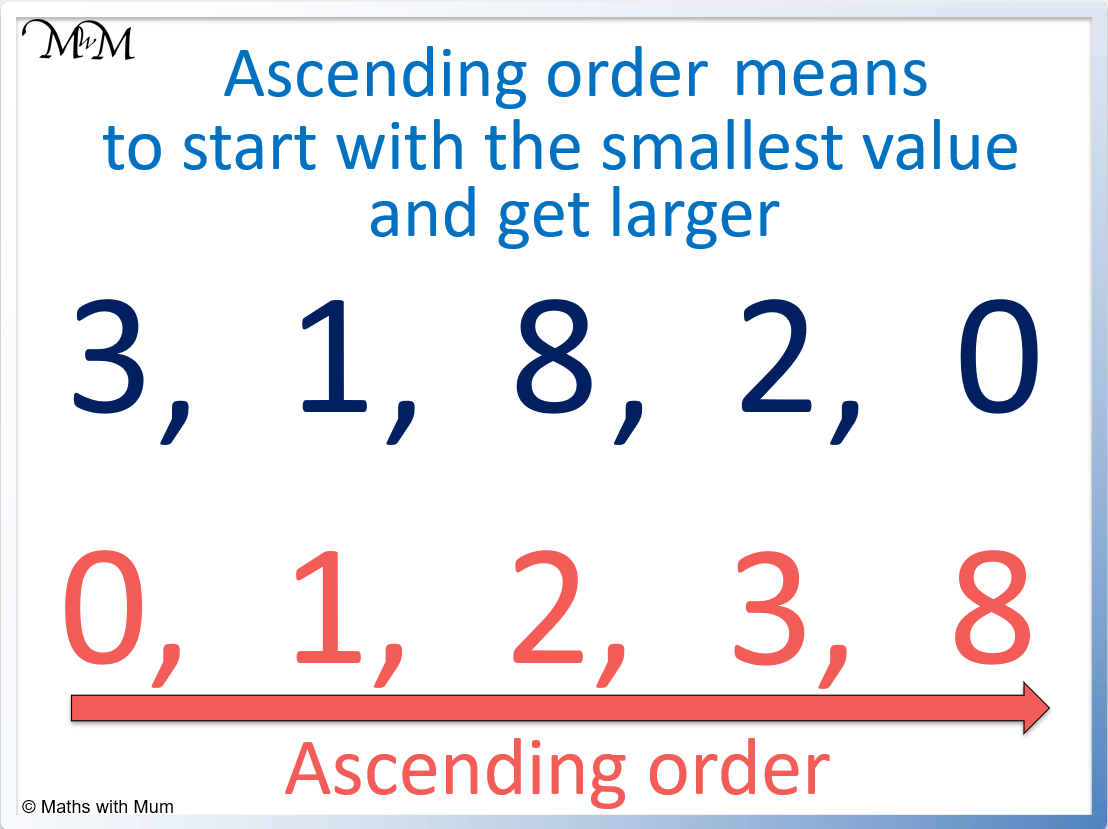• The numbers 3, 1, 8, 2, 0 written in ascending order are 0, 1, 2, 3, 8.
• Zero is smaller than all positive numbers.
• The symbol ‘<' means 'less than'.
• We can write numbers in ascending order like so: 0 < 1 < 2 < 3 < 8.Supporting Lessons

#### Ascending and Descending Order: Video Lesson# Ascending and Descending Order

## What is Ascending Order

Numbers written in ascending order are arranged from smallest to largest. For example, the numbers 3, 4, 1, 5, 2 written in ascending order are 1, 2, 3, 4, 5. The numbers increase in size.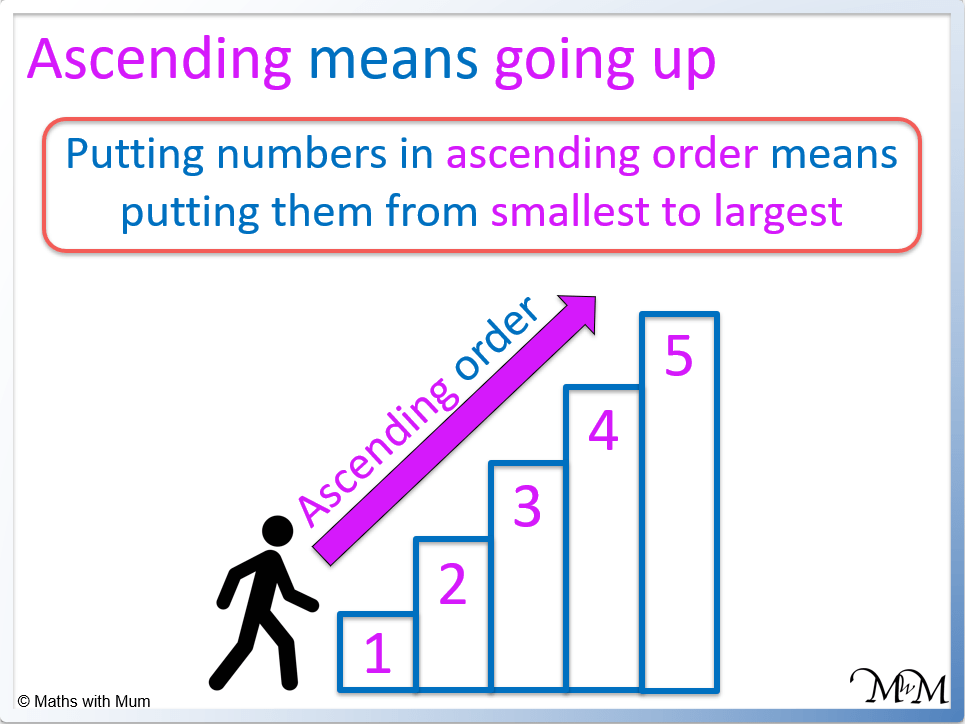Ascending means to go up or increase.Numbers can be written in ascending order using the less than symbol ‘<'. The smaller number is to the left of the sign and the larger number is to the right of the sign. For example, the numbers 1<2<3<4<5<6<7<8<9 are written in ascending order.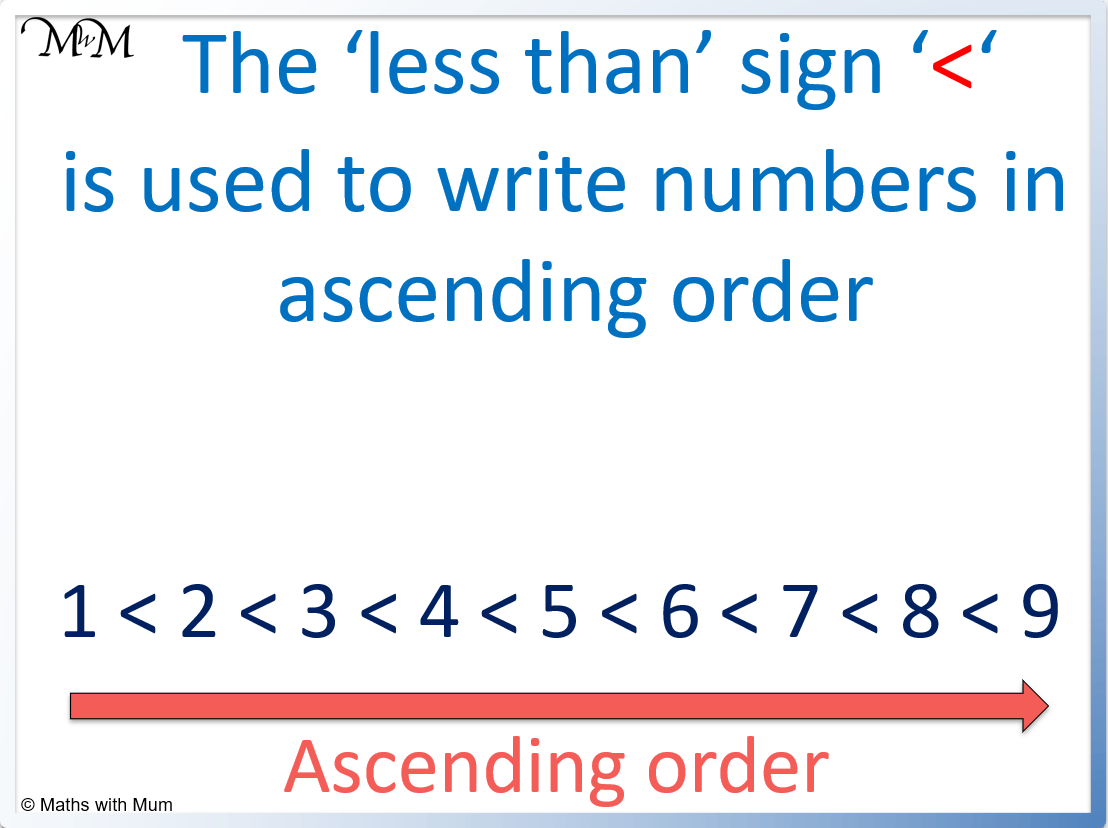Examples of numbers written in ascending order:

• 0 < 1 < 2 < 3 < 4 < 5
• 10 < 11 < 12 < 13 < 14 < 15
• 10 < 20 < 30 < 40 < 50 < 60 < 70 < 80 < 90 < 100
• -10 < -9 < -8 < -7 < -6 < -5 < -4 < -3 < -2 < -1 < 0 < 1
• 0 < 1/10 < 1/5 < 1/4 < 1/3 < 1/2 < 3/4

Writing numbers in ascending order is useful because it allows for the organisation of data. When numbers are written in ascending order, similar values can also be grouped together and extreme values can be found. Putting numbers in ascending order is also necessary for many calculations such as finding the median or quartiles.

## What is Descending Order

Numbers written in descending order are arranged from largest to smallest. For example, the numbers 3, 4, 1, 5, 2 written in descending order are 5, 4, 3, 2, 1. The numbers decrease in size.Descending means to go down. Numbers written in descending order go down.

The ‘greater than’ sign ‘>’ is used to arrange numbers in descending order. For example the numbers 9>8>7>6>5>4>3>2>1 are written in descending order.Examples of numbers written in descending order:

• 5 > 4 > 3 > 2 > 1 > 0
• 15 > 14 > 13 > 12 > 11 > 10
• 100 > 90 > 80 > 70 > 60 > 50 > 40 > 30 > 20 > 10
• 1 > 0 > -1 > -2 > -3 > -4 > -5
• 3/4 > 1/2 > 1/3 > 1/4 > 0

## How to Write Fractions in Ascending Order

• For fractions with the same denominator, the larger the numerator, the larger the fraction.
• For fractions with the same numerator, the smaller the denominator, the larger the fraction.
• For all other fractions, find a common denominator and the larger the numerator, the larger the fraction.

For example, the fractions 1/5, 2/5, 3/5, 4/5, 5/5 are in ascending order.

The fractions have the same denominator and so, they are ordered using the value of their numerator. The larger the numerator, the larger the fraction.For example, the fractions 1/6, 1/5, 1/4, 1/3, 1/2 are in ascending order.

The fractions have the same numerator and so, they are ordered using their denominator. The smaller the denominator, the larger the fraction.For example, the fractions 1/6, 2/3, 1/2, 5/12 and 3/4 can be written in ascending order by finding equivalent fractions with the same denominator.We can write the fractions as 2/12, 5/12, 6/12, 8/12 and 9/12.

Now the fractions have the same denominator, they can be ordered by arranging them according to the size of their numerator.

The fractions in ascending order are 1/6, 5/12, 1/2, 2/3 and 3/4.

## How to Write Fractions in Descending Order

Writing fractions in descending order means to put them in order from largest to smallest. To arrange fractions in descending order, find equivalent fractions with common denominators and then order them by their numerators. If fractions have the same denominator, those with the larger numerator are larger fractions.

For example, write the fractions 11/20, 7/10, 4/5, 3/4 and 1/2 in descending order.

These fractions can be written to have a common denominator of 20.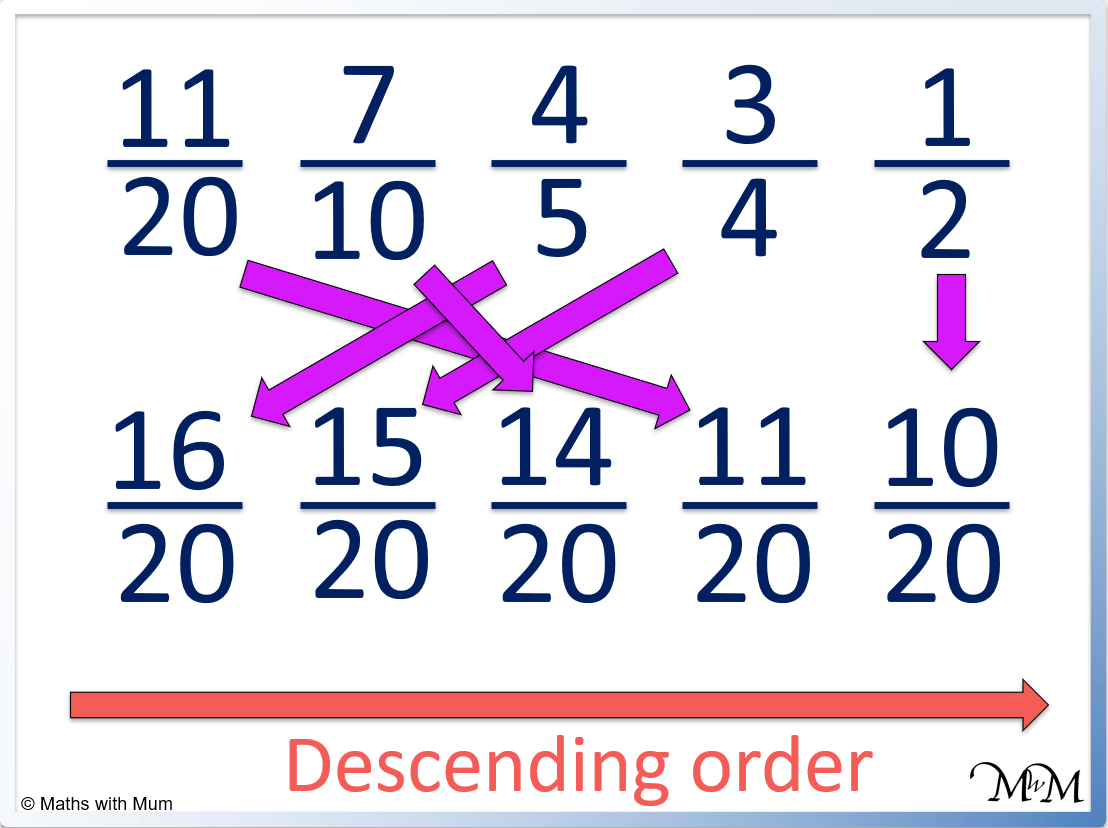The fractions in descending order are 4/5, 3/4, 7/10, 11/20 and 1/2.

## How to Write Decimals in Ascending Order

To arrange decimals in ascending order:

1. Compare each digit in each decimal from left to right.
2. The first decimal to have a larger digit is the larger decimal.
3. If the digits are the same, look at the next digit to the right.

For example, compare the size of the decimals 0.2 and 0.14.Starting from the left, both decimals have a 0 in the ones column.

So we look at the next digit in the tenths column. 2 is larger than 1 and so 0.2 is larger than 0.14. Therefore 0.14 < 0.2.

In ascending order, we write 0.14, 0.2.

For example, compare 0.3215 and 0.3211.Starting with the leftmost digits, both decimals have a 0, then a 3, then a 2, then a 1. In the final column, 0.3215 has a 5, whilst 0.3211 has a 1.

Therefore 0.3215 is larger than 0.3211. We can write 0.3211 < 0.3215.

In ascending order we have 0.3211, 0.3215.

To write lists of decimals in ascending order, compare each decimal number to each of the other decimals and write them from smallest to largest.

For example, write the decimals 0.2, 0.12, 0.034 and 0.105.

Each decimal has a ones digit of 0, so we compare the next digit to the right.

0.2 has a 2 in the tenths column, which is larger than 1 or 0 and so, 0.2 is the largest decimal. 0.034 has a 0 in the tenths column and therefore it is the smallest decimal.

Comparing 0.12 and 0.105, 0.12 has a 2 in the next column, whereas 0.105 has a 0. Therefore 0.12 is larger than 0.105.In ascending order, the decimals are 0.034, 0.105, 0.12 and 0.2.

## How to Write Decimals in Descending Order

Writing decimal in descending order means to write them from largest to smallest. To compare the size of decimals, compare each digit from left to right. The decimal with the largest digit is largest. If the digits are equal, compare the next digit to the right.

For example 0.09, 0.7, 1.02 and 0.11 written in descending order is 1.02, 0.7, 0.11 and 0.09.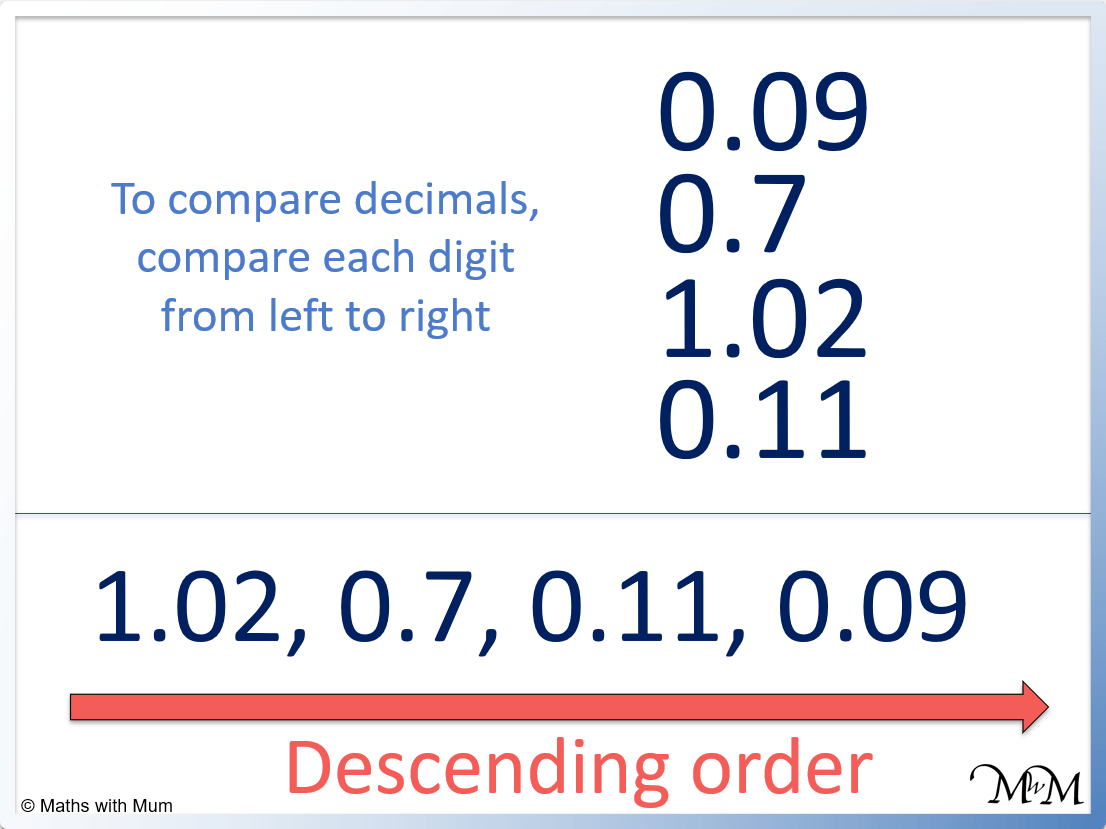## How to Write Negative Numbers in Ascending Order

To write negative numbers in ascending order, write them from smallest to largest. The bigger the number after the negative sign, the smaller it is. For example, 5 is bigger than 3 but -5 is smaller than -3. An example of negative numbers written in ascending order is -10, -8, -5, -3, -2, -1.Negative numbers written in ascending order appear backwards compared to positive numbers arranged in ascending order.The larger the number after the negative sign, the smaller the number.

Negative numbers are always smaller than positive numbers.

For example, the numbers -4, 5, 0, -2 and 1 arranged in ascending order are -4, -2, 0, 1, 5.

To write negative numbers in descending order, write them from largest to smallest. The larger the number after the negative sign, the smaller the number. For example, the numbers -1, -2 ,-3, -4, -5 are written in descending order.## Dates in Ascending Order

To write dates in ascending order, write the oldest dates first and the most recent dates last. For example in ascending order, the date of 01/01/1900 comes before 01/01/2020.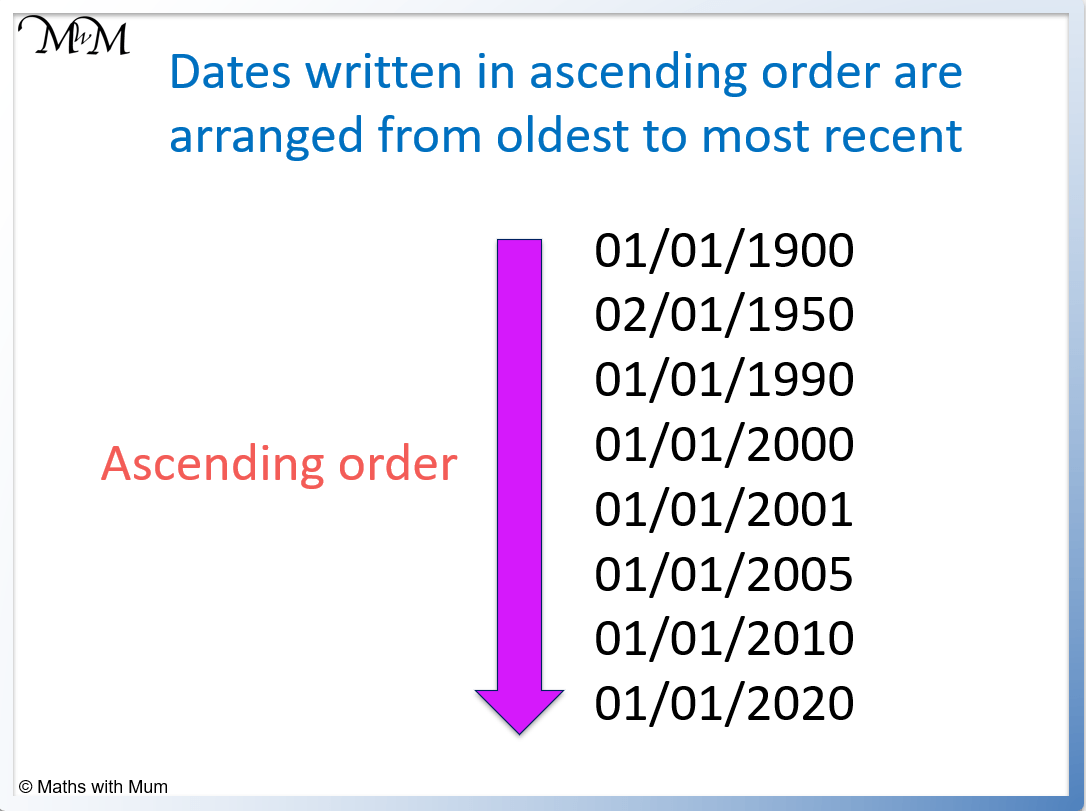## Dates in Descending Order

To write dates in descending order, write the most recent dates first and the oldest dates last. For example in descending order, the date of 01/01/2020 comes before 01/01/1900.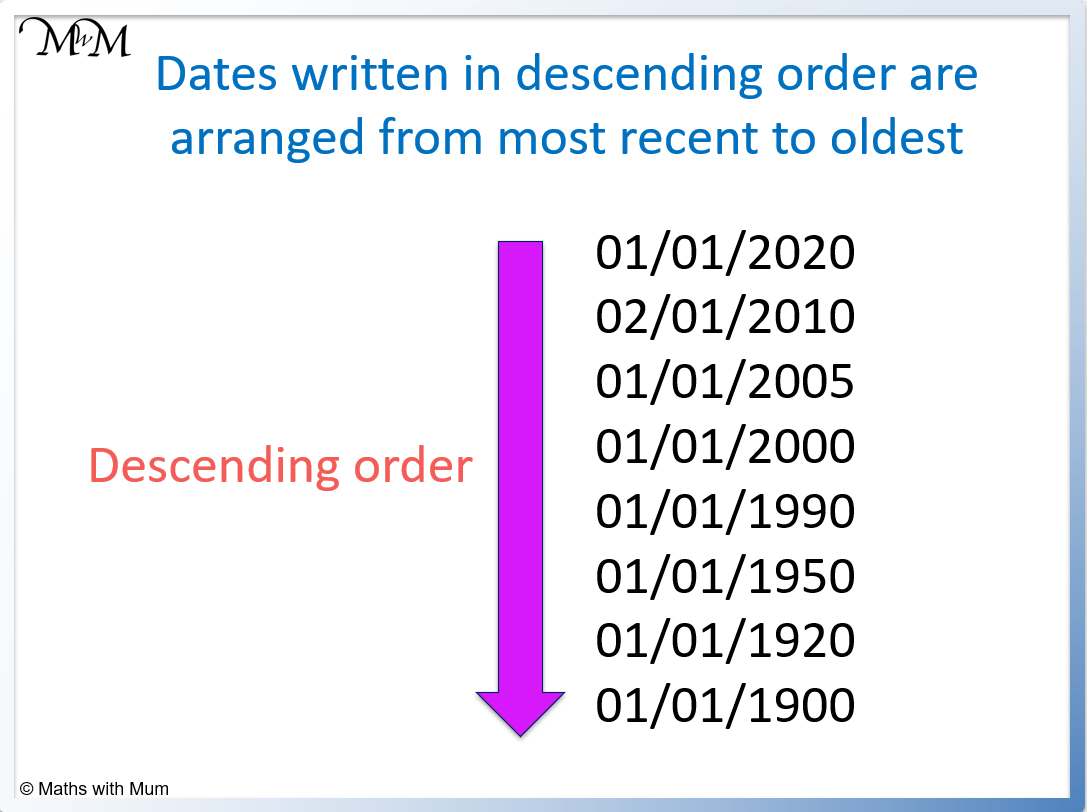## Ascending and Descending Alphabetical Order

Ascending alphabetic order sorts words by their first letter, with ‘A’ first and ‘Z’ last. Descending alphabetic order sorts the words with ‘Z’ first and ‘A’ last. Where words have the same first letter, the next letter is compared to order them.

• Ascending alphabetical order is from A to Z.
• Descending alphabetical order is from Z to A.

For example, the following words are arranged in ascending alphabetical order.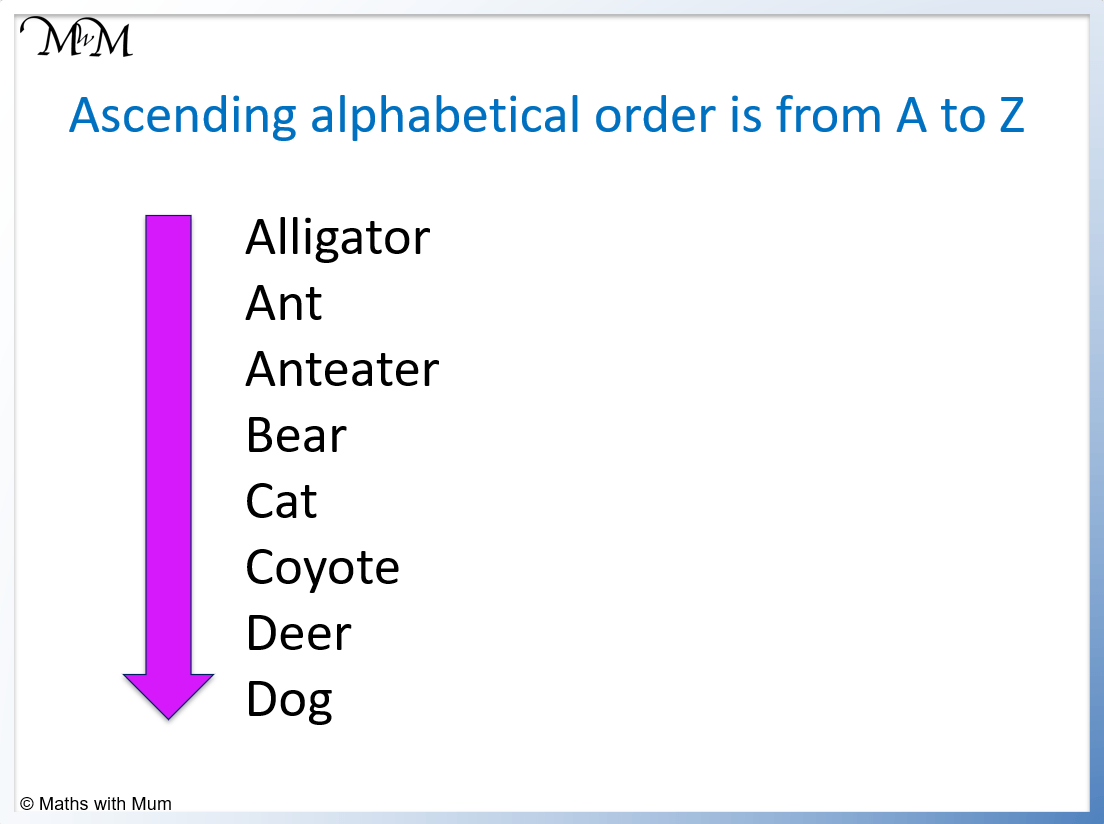Words in ascending alphabetical order: Alligator, Ant, Anteater, Bear, Cat, Coyote, Deer, Dog.

The following words are arranged in descending alphabetical order.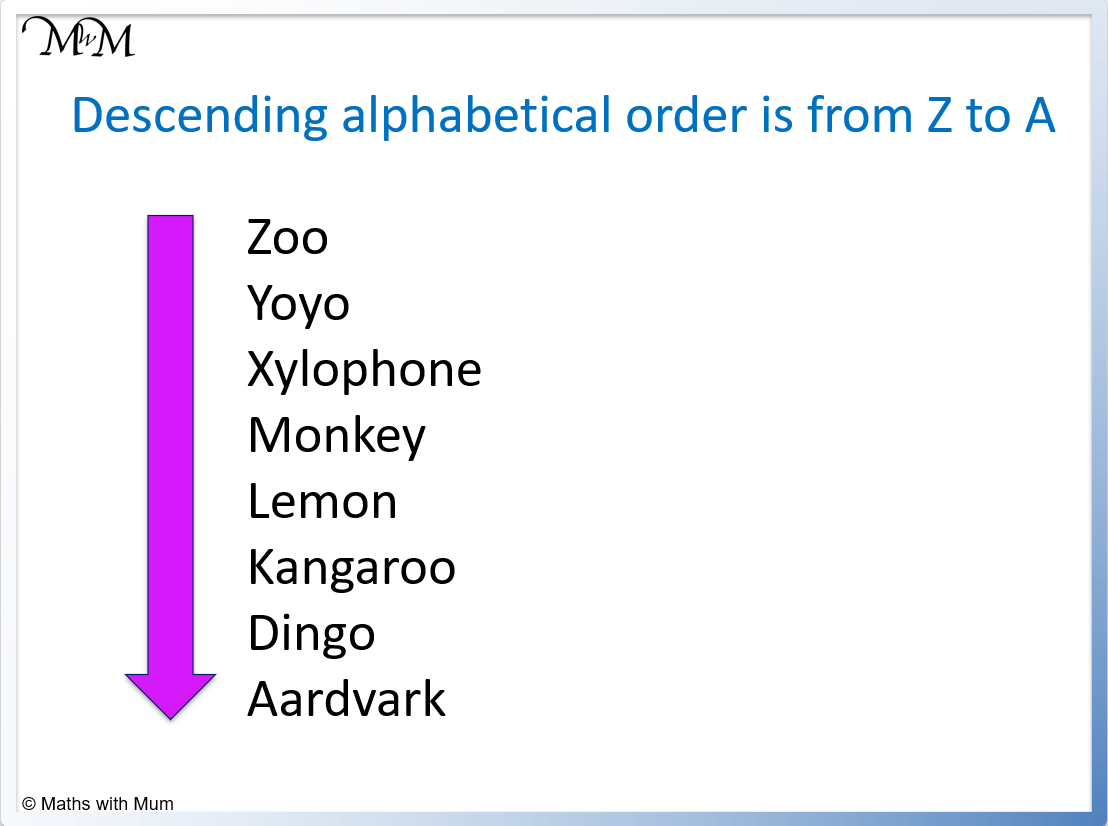Words in descending alphabetical order: Zoo, Yoyo, Xylophone, Monkey, Lemon, Kangaroo, Dingo, Aardvark.Now try our lesson on How to Compare Unlike Fractions where we learn how to compare fractions that have a different denominator.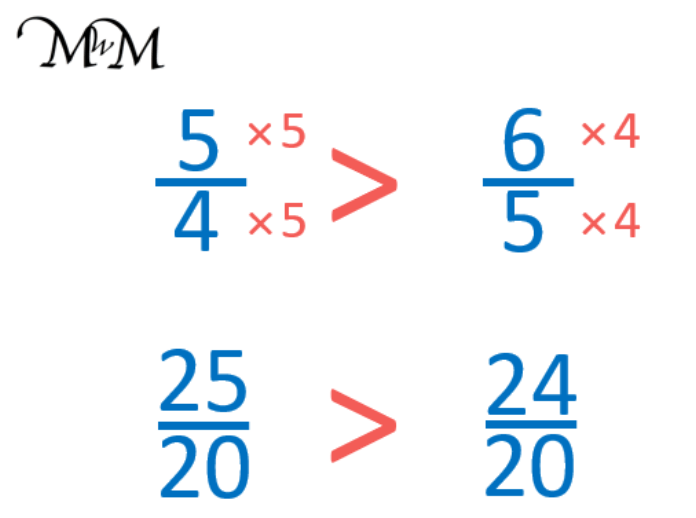error: Content is protected !!